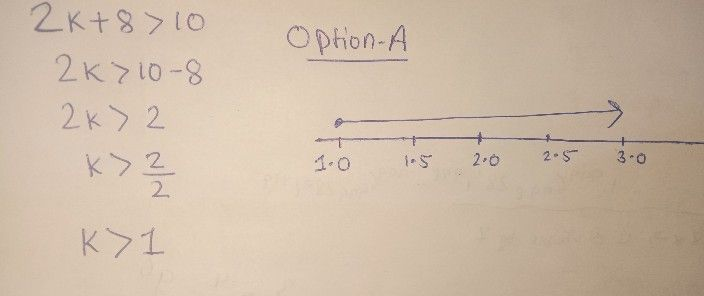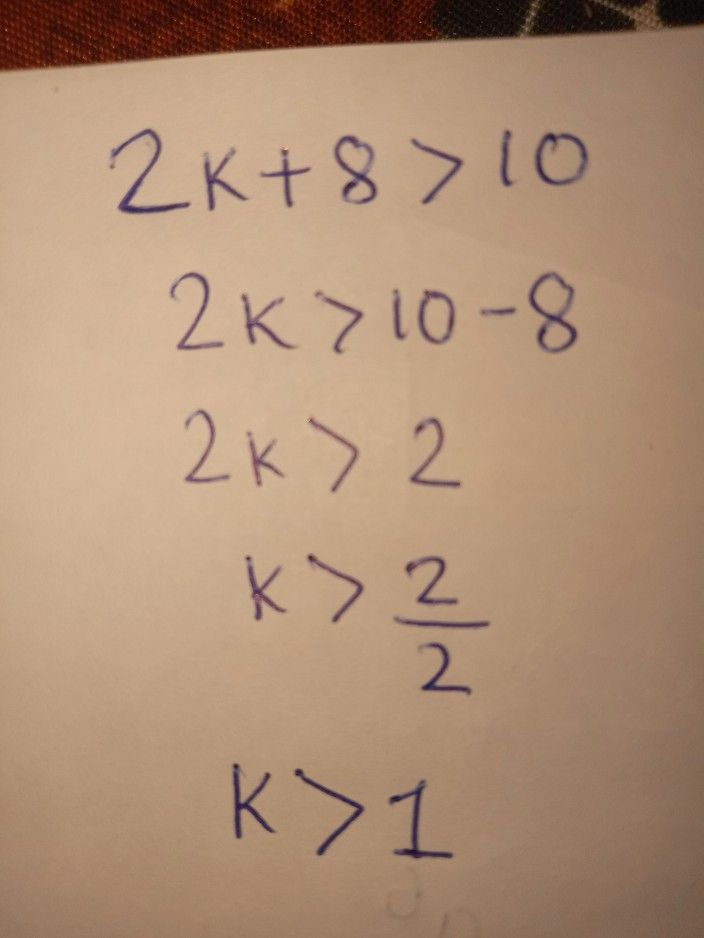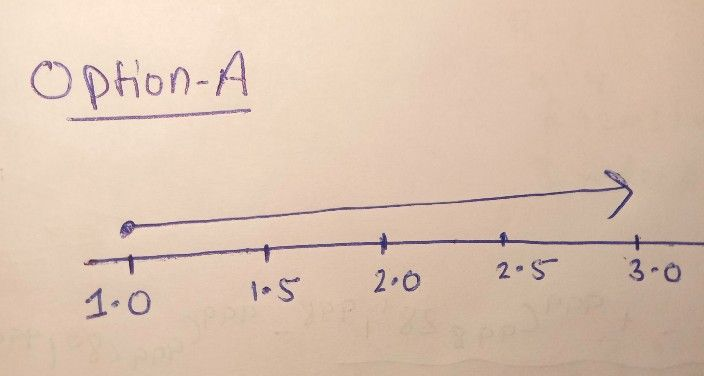Symbol
Problem$10.$ Simplify the following inequality, then graph it. $2k+8>10$ $○$ $1.0$ $1.5$ $2.0$ $2.5$ $3.0$ $○$ 10 $12$ $14$ $○$ 20 $25$ 30 $35$ $○$ $-6$ $-4$ $-2$
7th-9th grade
Algebra
SolutionQanda teacher - kavita❤️option (A)Student
Can you please explain a little bit more?Qanda teacher - kavita❤️
Solved the inequality hereValue of K will be greater then 1Student
Thank youQanda teacher - kavita❤️
and this is graph of thatStudent
Ok thanks